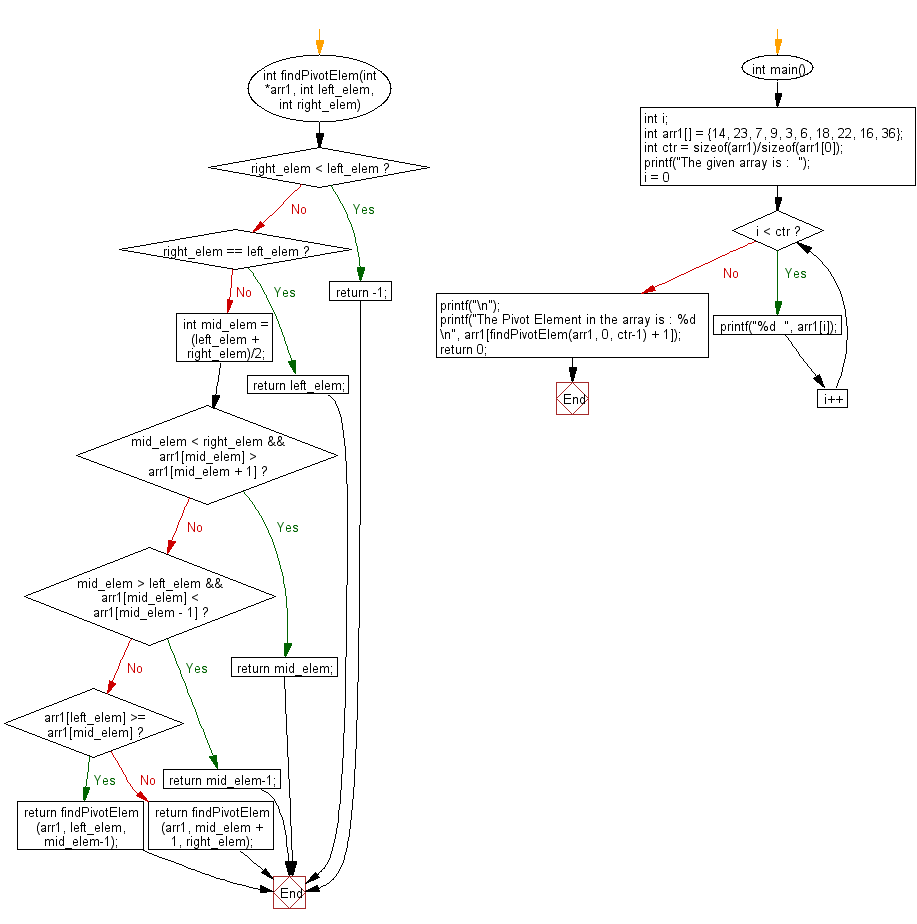﻿ C exercises: Find the pivot element of a sorted and rotated array using binary search - w3resource# C Exercises: Find the pivot element of a sorted and rotated array using binary search

## C Array: Exercise-37 with Solution

Write a program in C to find the pivot element of a sorted and rotated array using binary search.
Pivot element is the only element in input array which is smaller than it's previous element.
A pivot element divided a sorted rotated array into two monotonically increasing array.

Sample Solution:

C Code:

``````#include <stdio.h>

int findPivotElem(int *arr1, int left_elem, int right_elem)
{
if (right_elem < left_elem)
return -1;
if (right_elem == left_elem)
return left_elem;
int mid_elem = (left_elem + right_elem)/2;
if (mid_elem < right_elem && arr1[mid_elem] > arr1[mid_elem + 1])
return mid_elem;
if (mid_elem > left_elem && arr1[mid_elem] < arr1[mid_elem - 1])
return mid_elem-1;
if (arr1[left_elem] >= arr1[mid_elem])
{
return findPivotElem(arr1, left_elem, mid_elem-1);
} else
{
return findPivotElem(arr1, mid_elem + 1, right_elem);
}
}
int main()
{
int i;
int arr1[] = {14, 23, 7, 9, 3, 6, 18, 22, 16, 36};
int ctr = sizeof(arr1)/sizeof(arr1);
printf("The given array is :  ");
for(i = 0; i < ctr; i++)
{
printf("%d  ", arr1[i]);
}
printf("\n");
printf("The Pivot Element in the array is : %d \n", arr1[findPivotElem(arr1, 0, ctr-1) + 1]);
return 0;
}
```
```

Sample Output:

```The given array is :  14  23  7  9  3  6  18  22  16  36
The Pivot Element in the array is : 3
```

Flowchart :C Programming Code Editor:

Improve this sample solution and post your code through Disqus.

What is the difficulty level of this exercise?

﻿